# Parallel Wave Propagation

Consider wave propagation, at arbitrary frequencies, parallel to the equilibrium magnetic field. When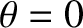, the eigenmode equation (5.42) simplifies to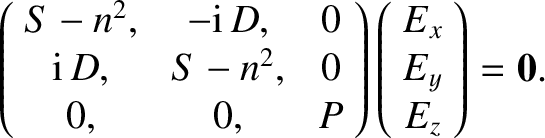(5.88)

One obvious way of solving this equation is to have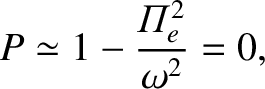(5.89)

with the eigenvector. This is just the electrostatic plasma wave that we found previously in an unmagnetized plasma. This mode is longitudinal in nature, and, therefore, causes particles to oscillate parallel to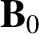. It follows that the particles experience zero Lorentz force due to the presence of the equilibrium magnetic field, with the result that this field has no effect on the mode dynamics.

The other two solutions to Equation (5.88) are obtained by setting the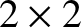determinant involving the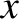- and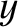-components of the electric field to zero. The first wave has the dispersion relation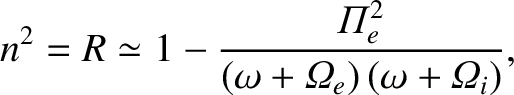(5.90)

and the eigenvector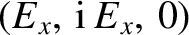. This is evidently a right-handed circularly polarized wave. The second wave has the dispersion relation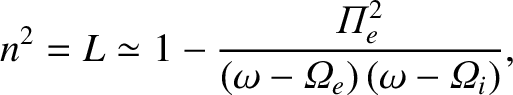(5.91)

and the eigenvector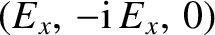. This is evidently a left-handed circularly polarized wave. At low frequencies (i.e.,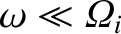), both waves convert into the Alfvén wave discussed in the previous section. (The fast and slow Alfvén waves are indistinguishable for parallel propagation.) Let us now examine the high-frequency behavior of the right- and left-handed waves.

For the right-handed wave, because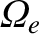is negative, it is evident that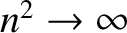as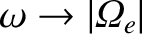. This resonance, which corresponds to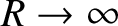, is termed the electron cyclotron resonance. At the electron cyclotron resonance, the transverse electric field associated with a right-handed wave rotates at the same velocity, and in the same direction, as electrons gyrating around the equilibrium magnetic field. Thus, the electrons experience a continuous acceleration from the electric field, which tends to increase their perpendicular energy. It is, therefore, not surprising that right-handed waves, propagating parallel to the equilibrium magnetic field, and oscillating at the frequency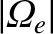, are absorbed by electrons.

When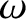lies just above, we find that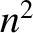is negative, and so there is no wave propagation. However, for frequencies much greater than the electron cyclotron or plasma frequencies, the solution to Equation (5.90) is approximately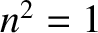. In other words,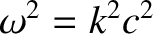, which is the dispersion relation of a right-handed vacuum electromagnetic wave. Evidently, at some frequency above, the solution formust pass through zero, and become positive again. Putting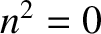in Equation (5.90), we find that the equation reduces to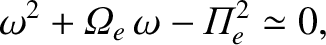(5.92)

assuming that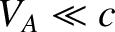. The previous equation has only one positive root, at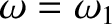, where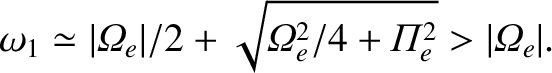(5.93)

Above this frequency, the wave propagates once again.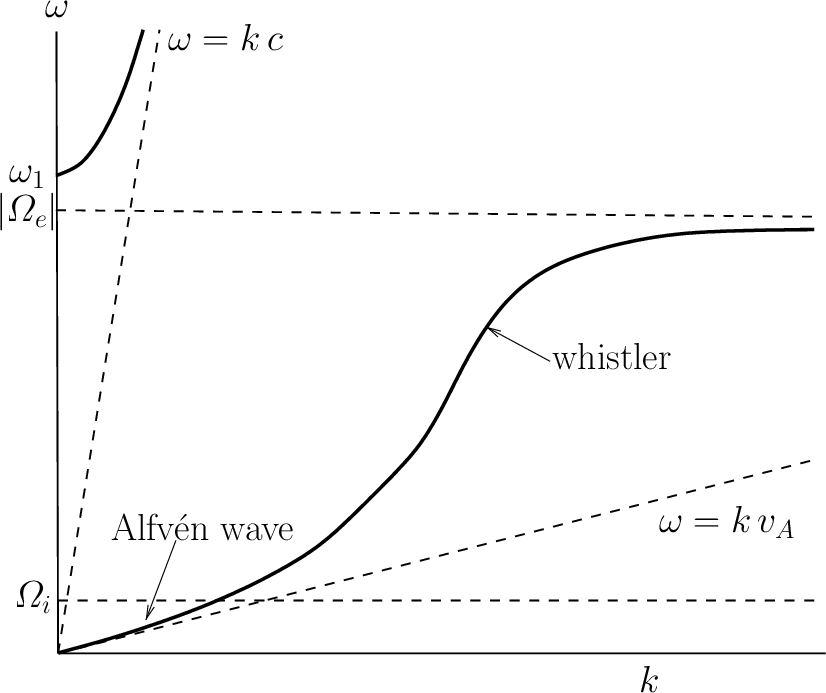The dispersion curve for a right-handed wave propagating parallel to the equilibrium magnetic field is sketched in Figure 5.1. The continuation of the Alfvén wave above the ion cyclotron frequency is called the electron cyclotron wave, or, sometimes, the whistler wave. The latter terminology is prevalent in ionospheric and space plasma physics contexts. The wave that propagates above the cutoff frequency,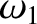, is a standard right-handed circularly polarized electromagnetic wave, somewhat modified by the presence of the plasma. The low-frequency branch of the dispersion curve differs fundamentally from the high-frequency branch, because the former branch corresponds to a wave that can only propagate through the plasma in the presence of an equilibrium magnetic field, whereas the latter branch corresponds to a wave that can propagate in the absence of an equilibrium field.

The curious name “whistler wave” for the branch of the dispersion relation lying between the ion and electron cyclotron frequencies is originally derived from ionospheric physics. Whistler waves are a very characteristic type of audio-frequency radio interference, most commonly encountered at high latitudes, which take the form of brief, intermittent pulses, starting at high frequencies, and rapidly descending in pitch.

Whistlers were discovered in the early days of radio communication, but were not explained until much later (Storey 1953). Whistler waves start off as “instantaneous” radio pulses, generated by lightning flashes at high latitudes. The pulses are channeled along the Earth's dipolar magnetic field, and eventually return to ground level in the opposite hemisphere. Now, in the frequency range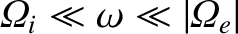, the dispersion relation (5.90) reduces to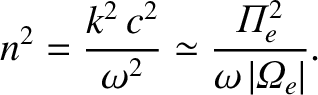(5.94)

As is well known, wave pulses propagate at the group-velocity,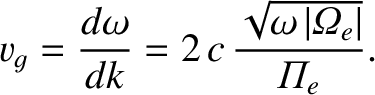(5.95)

Clearly, the low-frequency components of a pulse propagate more slowly than the high-frequency components. It follows that, by the time a pulse returns to ground level, it has been stretched out temporally, because its high-frequency components arrive slightly before its low-frequency components. This also accounts for the characteristic whistling-down effect observed at ground level.

The shape of whistler pulses, and the way in which the pulse frequency varies in time, can yield a considerable amount of information about the regions of the Earth's magnetosphere through which the pulses have passed. For this reason, many countries maintain observatories in polar regions—especially Antarctica—which monitor and collect whistler data.

For a left-handed circularly polarized wave, similar considerations to those described previously yield a dispersion curve of the form sketched in Figure 5.2. In this case,goes to infinity at the ion cyclotron frequency,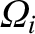, corresponding to the so-called ion cyclotron resonance (at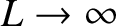). At this resonance, the rotating electric field associated with a left-handed wave resonates with the gyromotion of the ions, allowing wave energy to be converted into perpendicular kinetic energy of the ions. There is a band of frequencies, lying above the ion cyclotron frequency, in which the left-handed wave does not propagate. At very high frequencies, a propagating mode exists, which is basically a standard left-handed circularly polarized electromagnetic wave, somewhat modified by the presence of the plasma. The cutoff frequency for this wave is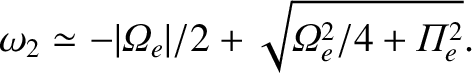(5.96)

As before, the lower branch in Figure 5.2 describes a wave that can only propagate in the presence of an equilibrium magnetic field, whereas the upper branch describes a wave that can propagate in the absence an equilibrium field. The continuation of the Alfvén wave to just below the ion cyclotron frequency is generally known as the ion cyclotron wave.